#### Risk Disclosure

Futures, foreign currency and options trading contains substantial risk and is not for every investor. An investor could potentially lose all or more than the initial investment. Risk capital is money that can be lost without jeopardizing ones financial security or lifestyle. Only risk capital should be used for trading and only those with sufficient risk capital should consider trading. Past performance is not necessarily indicative of future results. View full risk disclosure.

# Regression Channel Position Solver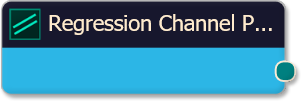The Regression Channel Position solver analyzes the relative position of price to the Regression Channel lines.  The Regression Channel is divided into 6 zones (A-F).  4 zones are inside the channel, and 2 zones are outside the channel.  Output Zones for the Long side start above the Upper channel line with Zone A and descend.  Output Zones for the Short side start below the Lower channel line with Zone A and ascend.  See the examples below for a visual explanation.

# BloodHound   v2

This section is specific to BloodHound v2.  Scroll down for BloodHound v1.

## Properties tab

This tab contains the essential settings for all nodes.

### Modifiers

Please note:   The buttons only modify the individual instance of the selected node(s) on a Logic Board.   Thus, the buttons are only visible when a node is selected on the Logic Board.  The buttons are not available when a solver is selected in the Solvers panel, because the original solver's output can not be globally modified.
This behavior is consistent with adding a function node afterwards, so that the original solver's output remains unmodified elsewhere in the system.  It is similar to having an SMA(50) on several charts.  Changing the plot color on one chart does not modify the plot color on the other charts.

### Global Properties

These setting apply to all instances of the selected solver.  Changing a setting in one of the instances changes the setting in all instances.  If you only want to change one of the solvers, but not the others, you must make a copy of the solver.  The copy will be a different solver, and a number will be added at the end of the name to differentiate it from the original.

### Behavior

This option determines which outputs are calculated.  Effectively turning off either the Long or Short output.

## Options tab

This tab contains extra secondary settings common to all nodes.

### Weight

This section is used to modify the node's final output value.  It is only useful for designing fuzzy logic systems, or a fuzzy logic section of a system.
Please note:   The Weight controls only modify the individual instance of the selected node(s) on a Logic Board.   Thus, the Weight controls are only visible when a node is selected on the Logic Board.  The controls are not available when a solver is selected in the Solvers panel, because the original solver's output can not be globally modified.
This behavior is different than BloodHound 1.0.  In BH 1.0, the Weight controls modified the nodes output globally (every instance).  This change in BH 2.0 adds more system design granularity.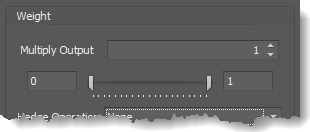Multiply Output:   This takes the internal values (the Long and Short values determined via the Properties tab » Output Rules section) and multiplies them by this value.  Note: the final output will not exceed a value of 1, as described in the Slider control below.

Slider control:  The slider constrains the final output to a value of 0 to 1.  The left side of the slider sets a minimum value that is output regardless if the solver condition is true or not.  The right side sets a maximum value that is output.  The output is capped.
e.g.  Three indicator conditions are being checked, and thus three solvers are created.  Only two out of the three indicator conditions are needed.  An Additive logic node is used to add the solver's outputs together.  Just two out of the three solvers need to add up to a value of 1.  Therefore, the right slider (max output value) for all three solvers is set to 0.5.  When two indicator/solver conditions are found, thus the outputs = 0.5, then the calculation, in the Additive node, is 0.5 + 0.5 + 0 = 1.  A value of 1 means the two out of three condition is true.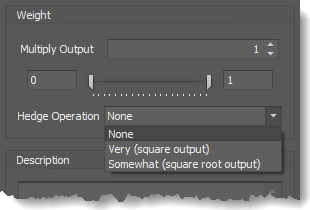Hedge Operation: This applies a mathematical formula to the internal value.

None:  No modification is applied.
Very (square output):  A squaring formula is applied.  Output  =  value .
Somewhat (square root output):  A square root formula is applied.  Output  =  √value .

### Description

This text area provides a place to write a full description of what the node is doing, used for, or what ever you want.
Note:   The Description is global to all instances of the node.  It is not applied individually to each instance as the Weight controls are.

## Help tab

This tab displays the documentation page (from this web site) of the selected node.
Please note:  NinjaTrader v8.0.26.0 or newer is required for the built in web viewer to work, and thus the documentation to be displayed.

### Video Tutorial

This video is from our weekly Workshop on Aug 22nd, 2012.
For more benefit please watch in full screen mode, as this video is recorded in HD.
This video shows BloodHound v1, however the settings and usage are the same.

### Examples

The screenshots below show BloodHound v1, however the settings and usage are still the same.

#### Example 1A: The Basics

This demonstrates the basic function of the Regression Channel Position solver, and how Long & Short Output Zones are inverted. When Zone B is set to 1 and price is between the upper quarter line and Upper channel line the Long output is 1. The reverse is true for the Short side, because the Output Zones are inverted. Therefore when price is between the lower quarter line and Lower channel line (Zone B for the Short side) the Short output is 1.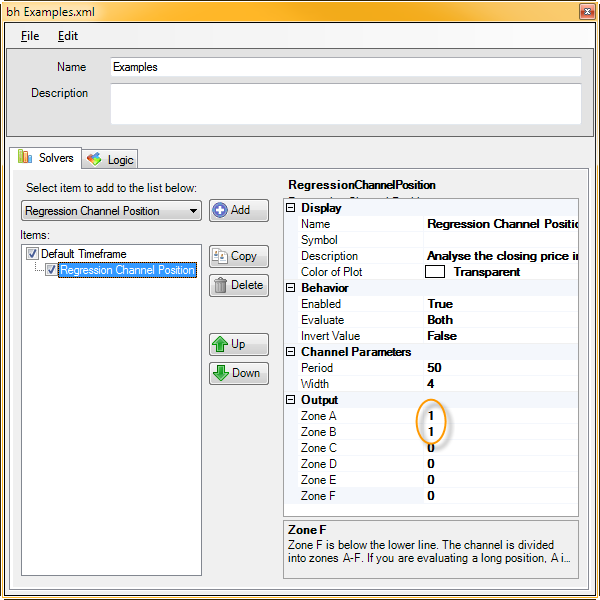• Add the Regression Channel Position solver
• Set Output Zone A & B to 1
• Set Output Zone C, D, E, & F to 0

As seen in the top chart, when price enters the Long Output Zone B the solver outputs 1 to the Long side.  In the bottom chart you will notice that the Zones are reversed.  Thus, when price enters the Short Output Zone B the solver outputs 1 to the Short side.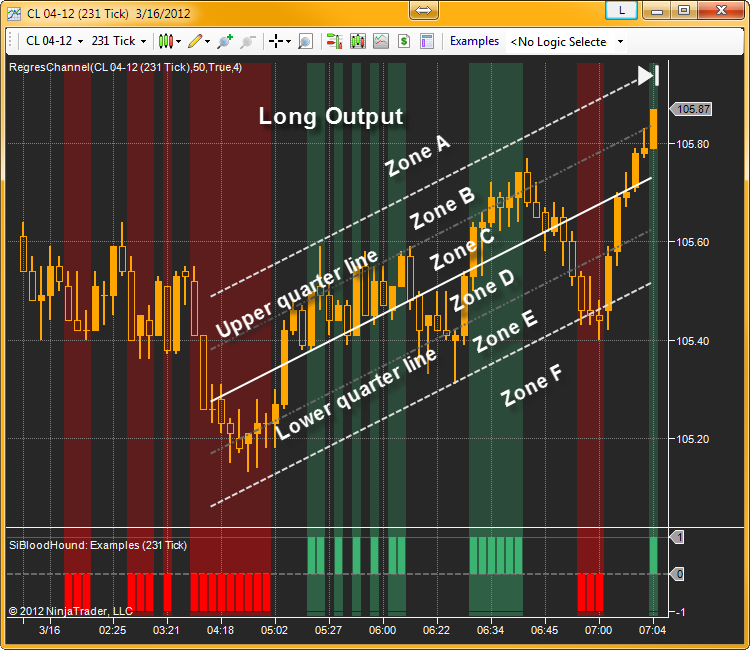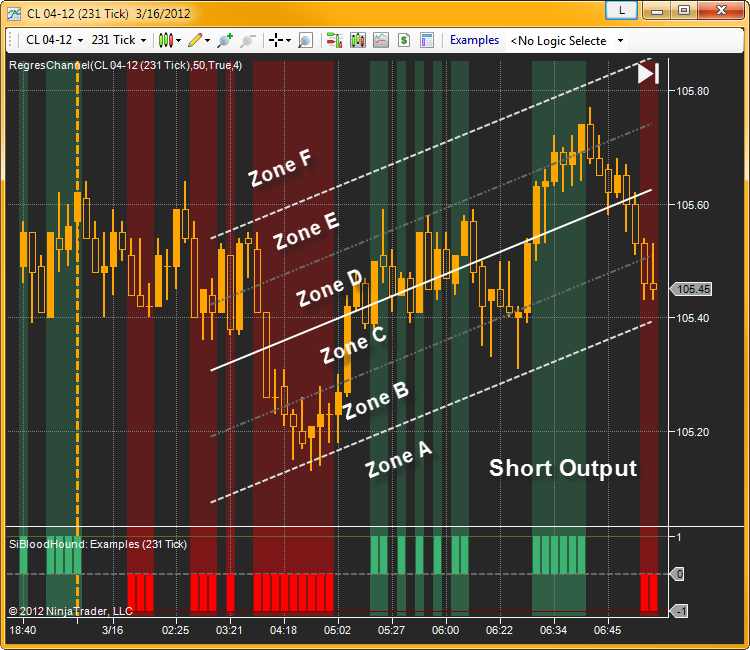#### Example 1B: The Basics

This second part will illustrate using the lower portion of the Output Zones.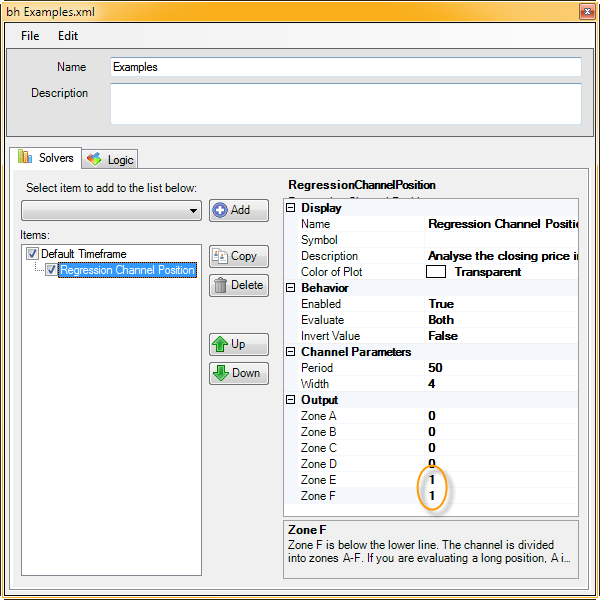• Add the Regression Channel Position solver
• Set Output Zone B, C, & D to 0

As seen in the top chart, when price enters the Long Output Zone E the solver outputs 1 to the Long side.  In the bottom chart, when price enters the Short Output Zone E the solver outputs 1 to the Short side.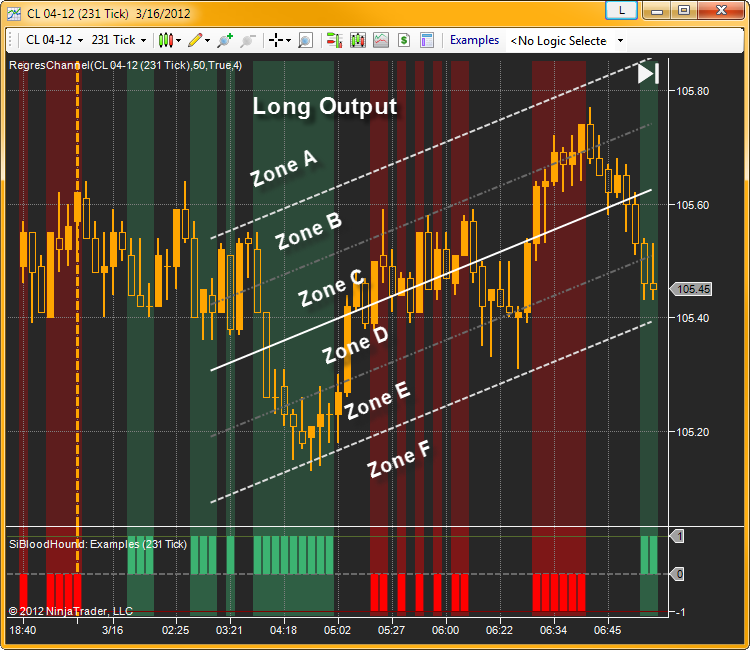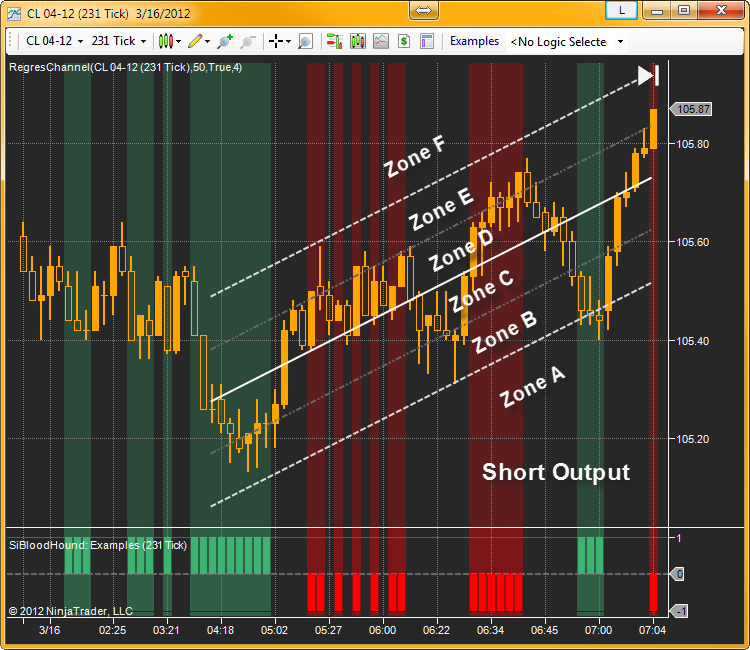#### Example 2: Channel Entry & Exit

In this example we will generate a Long signal when price is near the bottom channel line and the channel is sloping up, also a Short signal when price is near the upper channel line and the channel is sloping down.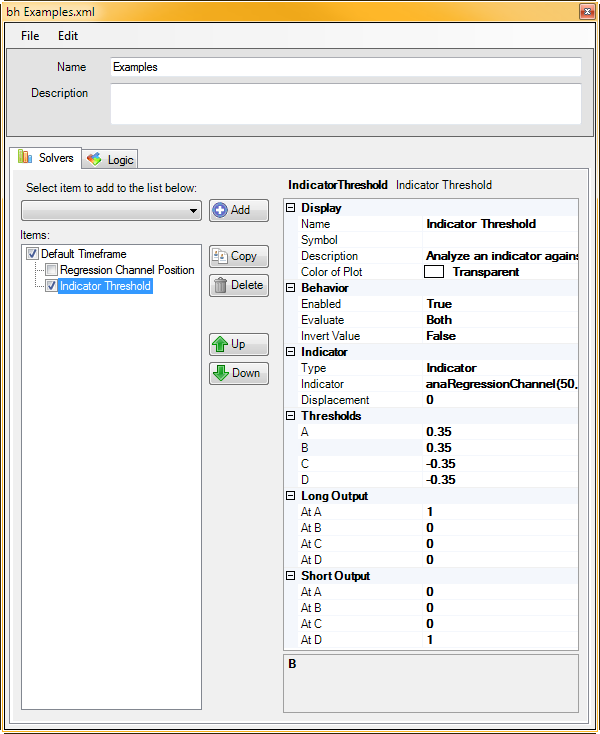• Add the Regression Channel Position solver, and use the setting as shown above
• Add the Indicator Threshold solver
• Set Thresholds A & B to 0.35
• Set Thresholds C & D to -0.35
• Set Long Output: At A to 1
• Set Short Output: At D to 1

Below we see two charts side by side, demonstrating the minimum slope requirement of the Regression Channel for a Long & Short output that the Indicator Threshold provides.  Note that the Regression Channel Position solver is not checked for this image.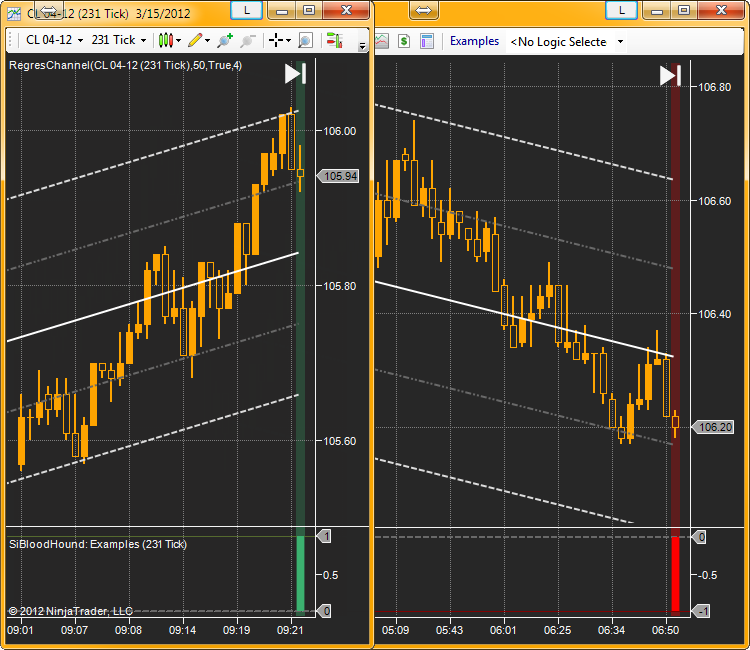With the Regression Channel Position solver checked, the charts below show signals when the minimum slope is obtained and price is near one of the outer channel lines.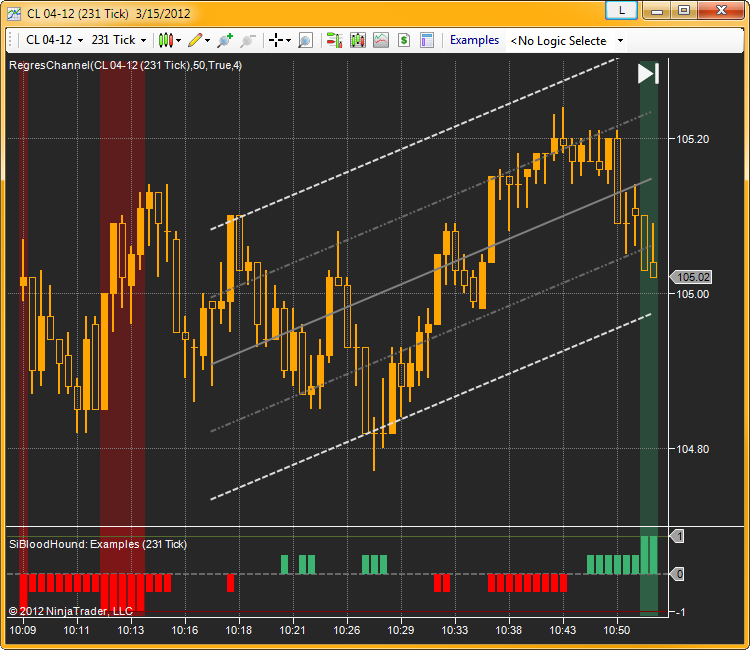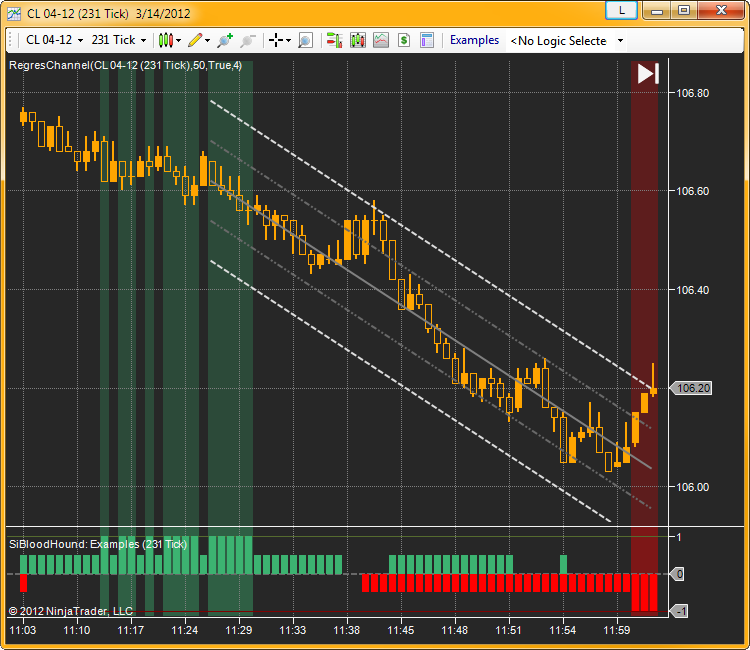#### Risk Disclosure

Futures, foreign currency and options trading contains substantial risk and is not for every investor. An investor could potentially lose all or more than the initial investment. Risk capital is money that can be lost without jeopardizing ones financial security or lifestyle. Only risk capital should be used for trading and only those with sufficient risk capital should consider trading. Past performance is not necessarily indicative of future results. View full risk disclosure.

# BloodHound   v1

This section is specific to BloodHound v1.  Scroll up for BloodHound v2.

# Regression Channel Position SolverThe Regression Channel Position solver analyzes the relative position of price to the Regression Channel lines.  The Regression Channel is divided into 6 zones (A-F).  4 zones are inside the channel, and 2 zones are outside the channel.  Output Zones for the Long side start above the Upper channel line with Zone A and descend.  Output Zones for the Short side start below the Lower channel line with Zone A and ascend.  See the examples below for a visual explanation.

### Video Tutorial

This video is from our weekly Workshop on Aug 22nd, 2012.
For more benefit please watch in full screen mode, as this video is recorded in HD.

### Examples

#### Example 1A: The Basics

This demonstrates the basic function of the Regression Channel Position solver, and how Long & Short Output Zones are inverted. When Zone B is set to 1 and price is between the upper quarter line and Upper channel line the Long output is 1. The reverse is true for the Short side, because the Output Zones are inverted. Therefore when price is between the lower quarter line and Lower channel line (Zone B for the Short side) the Short output is 1.• Add the Regression Channel Position solver
• Set Output Zone A & B to 1
• Set Output Zone C, D, E, & F to 0

As seen in the top chart, when price enters the Long Output Zone B the solver outputs 1 to the Long side.  In the bottom chart you will notice that the Zones are reversed.  Thus, when price enters the Short Output Zone B the solver outputs 1 to the Short side.#### Example 1B: The Basics

This second part will illustrate using the lower portion of the Output Zones.• Add the Regression Channel Position solver
• Set Output Zone B, C, & D to 0

As seen in the top chart, when price enters the Long Output Zone E the solver outputs 1 to the Long side.  In the bottom chart, when price enters the Short Output Zone E the solver outputs 1 to the Short side.#### Example 2: Channel Entry & Exit

In this example we will generate a Long signal when price is near the bottom channel line and the channel is sloping up, also a Short signal when price is near the upper channel line and the channel is sloping down.• Add the Regression Channel Position solver, and use the setting as shown above
• Add the Indicator Threshold solver
• Set Thresholds A & B to 0.35
• Set Thresholds C & D to -0.35
• Set Long Output: At A to 1
• Set Short Output: At D to 1

Below we see two charts side by side, demonstrating the minimum slope requirement of the Regression Channel for a Long & Short output that the Indicator Threshold provides.  Note that the Regression Channel Position solver is not checked for this image.With the Regression Channel Position solver checked, the charts below show signals when the minimum slope is obtained and price is near one of the outer channel lines.#### Risk Disclosure

Futures, foreign currency and options trading contains substantial risk and is not for every investor. An investor could potentially lose all or more than the initial investment. Risk capital is money that can be lost without jeopardizing ones financial security or lifestyle. Only risk capital should be used for trading and only those with sufficient risk capital should consider trading. Past performance is not necessarily indicative of future results. View full risk disclosure.

## Regression Channel Position SolverThe Regression Channel Position solver analyzes the relative position of price to the Regression Channel lines.  The Regression Channel is divided into 6 zones (A-F).  4 zones are inside the channel, and 2 zones are outside the channel.  Output Zones for the Long side start above the Upper channel line with Zone A and descend.  Output Zones for the Short side start below the Lower channel line with Zone A and ascend.  See the examples below for a visual explanation.

### Video Tutorial

This video is from our weekly Workshop on Aug 22nd, 2012.
For more benefit please watch in full screen mode, as this video is recorded in HD.

### Examples

#### Example 1A: The Basics

This demonstrates the basic function of the Regression Channel Position solver, and how Long & Short Output Zones are inverted. When Zone B is set to 1 and price is between the upper quarter line and Upper channel line the Long output is 1. The reverse is true for the Short side, because the Output Zones are inverted. Therefore when price is between the lower quarter line and Lower channel line (Zone B for the Short side) the Short output is 1.• Add the Regression Channel Position solver
• Set Output Zone A & B to 1
• Set Output Zone C, D, E, & F to 0

As seen in the top chart, when price enters the Long Output Zone B the solver outputs 1 to the Long side.  In the bottom chart you will notice that the Zones are reversed.  Thus, when price enters the Short Output Zone B the solver outputs 1 to the Short side.#### Example 1B: The Basics

This second part will illustrate using the lower portion of the Output Zones.• Add the Regression Channel Position solver
• Set Output Zone B, C, & D to 0

As seen in the top chart, when price enters the Long Output Zone E the solver outputs 1 to the Long side.  In the bottom chart, when price enters the Short Output Zone E the solver outputs 1 to the Short side.#### Example 2: Channel Entry & Exit

In this example we will generate a Long signal when price is near the bottom channel line and the channel is sloping up, also a Short signal when price is near the upper channel line and the channel is sloping down.• Add the Regression Channel Position solver, and use the setting as shown above
• Add the Indicator Threshold solver
• Set Thresholds A & B to 0.35
• Set Thresholds C & D to -0.35
• Set Long Output: At A to 1
• Set Short Output: At D to 1

Below we see two charts side by side, demonstrating the minimum slope requirement of the Regression Channel for a Long & Short output that the Indicator Threshold provides.  Note that the Regression Channel Position solver is not checked for this image.With the Regression Channel Position solver checked, the charts below show signals when the minimum slope is obtained and price is near one of the outer channel lines.